# Theoretical Background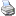backlinks... Cinderella Documentation Cinderella Handbuch Geometries 신데렐라 매뉴얼 Manual (Persian) Measurements Table of Contents Views English 日本語

# Theoretical Background

## Problems in Interactive Geometry

How should a system for doing interactive geometry behave when a user interacts with it? In a sense, the requirements are similar to the requirements for other programs:

• The program should be easy to use.
• The user should not be disturbed by unnatural behavior of the program.
• The user should not be tormented by being forced to make unnecessary input.
• The computed results should be correct.

Unfortunately, under these requirements interactive geometry turns out to be a difficult subject. There are two main reasons for this:

• There are problems that come from special cases that occur even in a static setup.
• There are problems that are of a genuinely dynamic nature.

### Static Problems

Our usual "everyday geometry" is full of special cases. Two lines can intersect or they can be parallel. Two circles can intersect in one or two points or not at all. So, even for static constructions it is sometimes difficult to figure out what a correct and reasonable result for such a special case should be. For instance, what is the angular bisector of two parallel lines? Is it undefined? Can it be any line parallel to the two lines? Should it be a line that is equidistant to the two lines?

We could try to exclude all the special cases and not allow them at all. However, on the one hand, this would mean excluding nonesoteric cases such as parallel lines. On the other hand, when we allow points to be moved in a construction, it happens all the time that mutually dependent elements are forced into special cases. Observe that this is still a static problem!

Such static problems have been studied for a long time. The great geometers of the nineteenth century were aware of them, and it is due to their effort that most of them could be solved. The key to a solution is gradually to extend Euclidean geometry to a larger domain. First, the usual plane is extended by elements at infinity, leading to projective geometry. Then the underlying algebraic structure is extended to cover complex numbers. This essentially removes all special cases from geometry.

It was an exciting development in mathematics when finally, around 1870, these approaches led to a completely consistent system. This system explains the effects of Euclidean geometry as well as those of non-Euclidean geometries, such as hyperbolic geometry. Today it is called Cayley-Klein geometry in honor of two of its main contributors.

The mathematical background and the implementation of Cinderella rely on this general setup. In this way, Cinderella can deal with all special cases, and as an additional benefit it is able to do non-Euclidean as well as Euclidean geometry. It is an amazing fact that by using these general principles the program does not become more complicated. On the contrary, the exclusion of special cases allows a much simpler and straightforward program structure.

### Dynamic Problems

For systems of interactive geometry there is a second class of problems, which are in a sense more subtle than the static problems. Unfortunately, they lead to even more drastic effects. Assume that you have created a construction that involves points, lines, and circles, and in particular the intersection of two circles (or of a circle and a line). When you move the mouse, the program has to decide, for every position, where the dependent elements are. However, there is a problem. Two circles do not have only one intersection. They have two, and we get both from our calculations. How should the system decide which of them is the one you "want"? When you construct the intersection, the answer to this question is easy: Take the point that is closest to the current mouse position. But when you start to move the construction, there is no obvious answer.

What would be most desirable is a continuous behavior of the program in the following sense:
If you make a very small move with a free point, then the elements that depend on it should also move only a little bit.

At first sight it is not clear whether this requirement is satisfiable in general. Turn on your favorite system for doing interactive geometry or parametric CAD and make the following experiment: Draw a horizontal line and construct two circles of equal radius whose centers are constrained to slide along the line. Move the circles to a position in which they intersect, and construct the upper point of intersection of the two circles. Now move one circle so that its center passes through the center of the other circle. Most probably you will see that the point of intersection suddenly jumps from the upper intersection to the lower one. This is what has happened in all the systems we have tried so far. Such behavior runs counter to our requirement of continuity: You make a small move, and a dependent point suddenly jumps.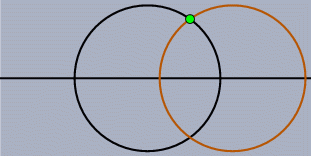This should not happen!

At first, a single jumping point seems to be a mere curiosity that is tolerable. But what happens if large parts of a construction depend on this jumping point? Then these parts of the construction will jump too, without prior warning. Most systems for interactive geometry use heuristics based on orientation decisions that help to get rid of some of these jump situations. But still, in every system many cases remain unresolved. In fact, there is a proof that no heuristic based on orientations only will be able to resolve all of these dynamic problems [Kor99], [KRG01], [RGK02]. In an article on dynamic geometry [Lab97], Jean-Marie Laborde, the main designer of Cabri Géométrie, states this dilemma in the following way:

I think we need a real mathematical treatment of all consequences of stretching geometry in some way to a wider (dynamic) system. This system cannot be the projective one if we want to maximize the way the environment takes into account the special characteristics of non-static objects which are at the core of dynamic geometry.

Cinderella is the first program that is based on a theory that is capable of preventing dependent elements from jumping. This theory is also based on the use of complex numbers, which were used to solve the problems of static geometry.

The use of this theory has many benefits. For instance, it is the basis of the generation of correct loci. Consider the Three-Bar Linkage example of the second tutorial. The generation of the locus is based on the correct calculation of the points of intersection of two circles while a free point moves. In other systems for interactive geometry you will probably get only half of the figure-eight curve. The methods for automatic theorem proving, which are used internally throughout Cinderella, are based on this theory as well.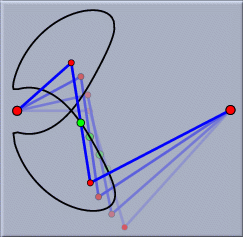The "three-bar locus" revisited

The following paragraphs should give you an impression of the different mathematical methods and theories that form the basis of the implementation of Cinderella.

## Projective Geometry

The first and perhaps most important step for a consistent geometric setup is to extend the usual Euclidean plane to contain elements at infinity. You have likely heard the phrase "parallel lines meet at infinity," and you might believe it when looking from a bridge along a very long and straight railroad track. This phrase is the key to projective geometry. The extension of geometry by infinite elements removes many of the special cases from usual Euclidean geometry.

Projective geometry has a very long tradition. Its historical origin can be traced back to the study of perspective undertaken by famous painters such as Albrecht Dürer and Leonardo da Vinci. Its mathematical origin is the work of Gaspard Monge, a French geometer, who developed, around 1795, a method called descriptive geometry for representing spatial configurations by planar perspective drawings. Monge observed that nontrivial facts about planar geometric configurations could be derived by considering these configurations as projections of configurations in space. The study of parallels in these projections was most elegantly accomplished by extending the plane by elements at infinity.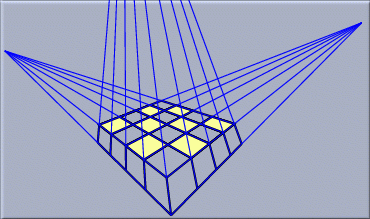In perspective drawings, parallels actually meet

The projective plane consists of the points of the usual, Euclidean, plane together with one additional "infinite" point for every possible direction. The lines of the projective plane are the Euclidean lines together with one special "line at infinity." All infinite points lie on the line at infinity. The following nicely symmetric relations between points and lines hold:

• Every two distinct points have a unique connecting line (their join).
• Every two distinct lines have a unique point of intersection (their meet).

The first person who formalized these rules, around 1822, was Victor Poncelet, a student of Monge, who today may be considered the "father of projective geometry." In projective geometry there is no need to consider parallels as something special. They still have a point of intersection; it just happens to lie at infinity. For a readable introduction to projective geometry we refer to the books of H. S. M. Coxeter on that topic [Cox49], [Cox63].

## Homogeneous Coordinates

On a computer we unfortunately do not have geometric objects as primitive data types. A point or a line has to be represented by numbers: the coordinates. Usually, a point in the plane is described by its (x, y) coordinates. A line may be given by the three parameters (a, b, c) of its defining equation ax + by + c = 0. However, when we want to do projective geometry, this turns out to be impractical. Each pair of coordinates (x, y) represents a finite point, and there is no representation for the points at infinity. A solution to this problem gradually became clear in the first half of the nineteenth century. It started with Möbius's barycentric coordinates, via the refined setup of homogeneous coordinates given by Plücker, and finally led to Grassmann's setup of multilinear algebra.

The way out of the dilemma is as follows. For every point we use three instead of two coordinates, thereby introducing a third dimension. Consider the following scenario: The plane is embedded parallel to the xy-plane of three-space at a height of z = 1, so it does not pass through the origin (0, 0, 0).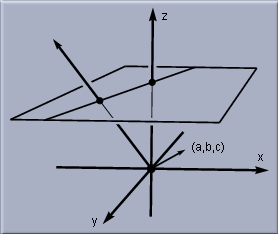Embedding the plane in space

Every point (x, y) is represented by its three-dimensional coordinates (x, y, 1). These coordinates are the homogeneous coordinates of the point. What happens with the rest of the points in the three-dimensional space? Almost all of them will be interpreted as points in the original plane: we identify all three-dimensional points that differ by a nonzero multiple. For instance, (4, 6, 2) and (2, 3, 1) describe the same point. In general, a point with spatial coordinates (x, y, z) is identified with the point (x/z, y/z, 1) of the original plane. This process is called dehomogenization. In a way, every point of the original plane corresponds to the line spanned by this point and the origin in three-space.

However, there are points in three-space that do not correspond to points in the original plane. The points of the form (x, y, 0) cannot be dehomogenized in the above way, since we would have to divide by zero. These points correspond precisely to the "points at infinity" of projective geometry. To see this, we study the behavior of a point that gradually moves to infinity in the original plane.

Assume that the moving point has coordinates (r, r). As r becomes larger and larger, this point gradually approaches a point at infinity in the 45º direction. Looking at its homogeneous coordinates, we see that they have the form (r, r, 1) ~ (1, 1, 1/r). As r increases, the contribution of the first two coordinates dominates the last coordinate more and more. In the limiting case of r equals "infinity," the homogeneous coordinates are given by (1, 1, 0), an infinite point. You can also try to imagine the line through this point and the three-dimensional origin. As the point approaches infinity, this line becomes more and more horizontal, until, in the limiting case, it is contained entirely in the xy-plane.

A similar representation can be given for lines. For the line ax + by + c = 0 we take the parameters (a, b, c) as the homogeneous coordinates of the line. As in the case of points, we identify nonzero multiples of such coordinates, since they do not alter the solution space of the corresponding equation. There is one set of parameters, (0, 0, 1), that does not correspond to a finite line. This is the line at infinity. The vector (a, b, c) of a line is orthogonal to the plane spanned by the corresponding line and the origin of three-space. In particular, the vector (0, 0, 1) is orthogonal to the xy-plane, the "line at infinity."

In fact, the algebraic notion of homogeneous coordinates provides a complete symmetry between points and lines. Each point or line is represented by three homogeneous coordinates. A point (x, y, z) lies on a line (a, b, c) if and only if the scalar product ax + by + cz is zero, which is simply rewriting the equation of the line. Geometrically, this means that the two corresponding vectors are orthogonal in three-space.

## Complex Numbers

It is not only geometry that has been extended throughout the centuries. A similar process happened to numbers. Probably the first numerical concept considered by mankind was that of the positive integers: 1, 2, 3, …. From that beginning, it was reasonable to extend the number system gradually to encompass more powerful concepts. The negative numbers, the rational numbers, and the real numbers had to be invented to achieve a useful and self-contained system. The observation that there must be numbers that cannot be represented as quotients of two integers is of a geometric nature and dates back to approximately 600 B.C.E. It was observed by the Pythagoreans that there is no rational number measuring the length of a diagonal of a square with sides of length one. Through application of the Pythagorean theorem, this task is equivalent to finding a number x such that x² = 2. This discovery led to a deep crisis in the foundations of ancient geometry.

However, the story of extending the number system does not stop at that point. One of the extensions, with perhaps the most drastic consequences, was the introduction of complex numbers. It was Geronimo Cardano in his 1545 work Ars Magna who was first to explicitly propose such an extension of the real numbers. He was led to his conclusions by the study of solutions of polynomials of degree three. Based on the work of other contemporary mathematicians, he discovered that a complete systematic representation of these solutions can be given only with the help of hitherto unknown values involving square roots of negative numbers.

A complex number is a number of the form a + i·b, where the symbol i satisfies the equation i² = –1, and a and b are real numbers. Clearly, the number i cannot be a real number, since the square of a real number can never be negative. The system of complex numbers is, like the real numbers, closed under the four basic arithmetic operations of addition, subtraction, multiplication, and division (excluding, of course, division by zero). In other words, the sum and the product of two complex numbers, as well as their difference and quotient, can again be written in the form a + i·b for suitable parameters a and b. However, unlike the real numbers, the system of complex numbers is also closed under the operation of finding solutions of polynomials. For instance, consider the polynomial

 x² – 6x + 13 = 0.

As you can easily check, it has no real solutions, but the complex numbers 3 + 2i and 3 – 2i do solve this equation. In fact, the following beautiful result is true: Every polynomial equation with arbitrary real or complex coefficients has all its solutions again in the field of complex numbers.

In a sense, the discovery of complex numbers is the starting point for most modern mathematics. Many mathematical theories find their broadest, most elegant, and most economical setting when they are formulated over the complex numbers. This happens also with geometry. Consider the situation of two circles. Depending on their position, they can have two, one, or no points of intersection.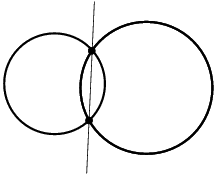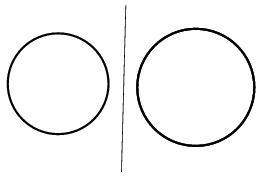Circles can intersect… …or not.

Finding the coordinates of the points of intersection amounts to solving a quadratic equation. Over the real numbers, this equation might have no solution. In this case, the circles do not intersect. Over the complex numbers, however, a solution always exists. So in the case of visually nonintersecting circles, we say that the intersections still exist, but that they have complex coordinates, and therefore we cannot see them in the real plane.

Cinderella's mathematical kernel is implemented entirely over the complex numbers. So when intersections visually vanish, Cinderella does not have to deal with special cases, and it can still continue calculating. The solutions just have complex coordinates.

What happens if two complex points are connected by a line? In general, this line will also have complex coordinates. However, if the points are so-called complex conjugates, which means that they differ only by the sign of their complex part, then their join is again real. Since the solutions of a quadratic equation with real coefficients are always complex conjugates, it follows that the intersection points of two circles are always complex conjugates. This is the reason why the line joining them is a real line, no matter where in the plane the circles are located, even if the intersection points are complex and therefore invisible. Cinderella will correctly calculate and draw this line, independent of the position of the circles. It may take a while to get used to the fact that intermediate results can disappear while some constructions depending on them remain visible. However, this is exactly what you should expect. Consider the case in which the circles have the same radius. The line is then the perpendicular bisector of the segment joining the two midpoints. If you include the complex situations, you are not compelled to consider so many special cases.

Another example of a theorem in which intermediate steps disappear is the following statement about three circles. Construct the line joining the two points of intersection of each pair of circles. The three chords that you obtain in this way meet in a point, no matter whether the circles intersect or not.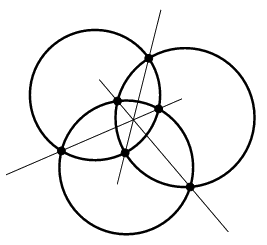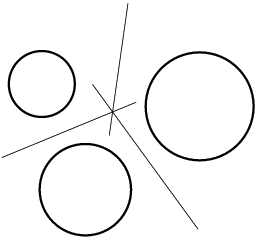Intermediate points can be complex… …but theorems remain true!

So in Cinderella each point and each line is represented by complex homogeneous coordinates. This means that altogether, any point or line has a six-dimensional(!) internal representation in the mathematical kernel. This may sound crazy, but it is the most natural thing to do.

## Measurements and Complex Numbers

If we were satisfied with projective incidence theory, then the system presented so far would be fairly complete. However, we want to be able to measure distances and angles, too. Measurements are in a sense the most fundamental geometrical operations. Unfortunately, at first glance, projective geometry does not appear to be capable of measuring, since under perspective transformations distances can change. Actually, for a long time mathematicians considered projective geometry a "nice toy" for doing incidence geometry, but not appropriate for the real stuff: measuring.

History proved them wrong. With the right setup, projective geometry is the universal system for doing measurements. This system unifies and explains different kinds of measurements. For example, it explains the relationship between Euclidean and hyperbolic geometry. However, it took a long time to finally find the algebraic setting in which Projective Geometry develops its full power. The key objects are called "Cayley-Klein geometries" in modern terms. It is an elegant and consistent mathematical approach to measurements that combines projective geometry and complex numbers.

### Euclidean and Non-Euclidean Geometry

One part of this development started with the discovery of non-Euclidean geometries. Our everyday geometry is, with relatively great accuracy, described by Euclid’s five postulates. He used these postulates to axiomatize geometry. This happened almost 2000 years ago. The last postulate, the so-called parallel axiom, plays a special role in the development of geometry. A way to formulate it is this: "Whenever there is a line l in the plane and a point P not on l, then there is exactly one line through P that does not meet l."

Euclid was very cautious about using the parallel postulate. Large parts of Euclid’s elaborations, for instance the complete theory of congruence of triangles, were done without the explicit need for this axiom. Today we are relatively sure that Euclid himself believed that this axiom was a consequence of the other four axioms. But he could not prove this. After Euclid, many other mathematicians tried to do so, and some of them even presented proofs. But all these proofs were incorrect.

In the sixteenth to eighteenth centuries, mathematicians also found many equivalent formulations for the parallel postulate. One of the most prominent formulations is _"The interior angles in a triangle sum up to 180º."__ If this statement could be derived from Euclid's first four axioms, then this would prove the dependence of the parallel postulate.

Proving that an axiom is dependent can be done by assuming its contrary and drawing conclusions until a contradiction is shown. Many people tried this, among them C.F. Gauss, J. Bolyai, and N. Lobachevski. They drew conclusion after conclusion, but to their surprise, instead of arriving at a contradiction, they found themselves developing a beautiful geometric system: hyperbolic geometry. There Euclid's parallel postulate is modified in the following way: "Whenever there is a line l in the plane and a point P not on l, then there will be more than one line through P that does not meet l." A consequence of this assumption is that the angle sum in a triangle is always less than 180º. Between 1815 and 1824, independently from each other, these three people, who are today considered the discoverers of hyperbolic geometry, came to the point at which they declared their system free from contradictions, simply because they could not find any. The system they developed was full of inner beauty, and it is a surprising fact that they could prove that under the assumption of the perturbed parallel postulate they end up with a unique theory (up to trivial isomorphisms).

It is worth mentioning that most probably Gauss was the first to arrive at these conclusions, around 1816. However, he did not dare to publish his results, since he was afraid of conflicts with the leading schools of Kantian philosophy at that time. They considered a straight line as the first thing whose nature is a priori clear.

If you are interested in the history of mathematics, we want to point you to the books of Bell [Bel45a], [Bel45b] and Struik [Str87]. As an introduction to hyperbolic geometry we recommend the book of Greenberg [Gre74].

### Cayley-Klein Geometries

For a long time it was not clear whether the system of hyperbolic geometry was indeed free of contradiction. What was missing was a model of this structure, a mathematical object that satisfied Euclid's first four axioms and the perturbed parallel postulate. With minor flaws, Beltrami was the first to construct such a model in 1868. However, the full beauty of a general theory was first seen when Felix Klein, a student of Plücker, presented his version of what we call "Cayley-Klein geometries"; see, for instance, [Kle28b]. What he gave was essentially a reduction of hyperbolic geometry to constructions of Euclidean geometry that implies the following: "If Euclidean geometry is free of contradictions, then so is hyperbolic geometry." This finally solved all problems surrounding Euclid's fifth postulate.

The idea behind Cayley-Klein geometries is to use the projective plane, and to distinguish a special conic, the "fundamental object." A special kind of global measurement is defined that depends only on the fundamental object. Depending on the type of the fundamental object you have chosen, you get different types of geometries: Euclidean geometry, hyperbolic geometry, elliptic geometry, relativistic geometry, and three other geometries of minor importance.

We will not go into the details of Cayley-Klein geometries, but we will present the major definitions and demonstrate some basic effects. We first need the concept of a cross ratio: For four points A, B, C, and D on a line, the cross ratio is defined as the number

 (A, B | C, D) := ((A – C)(B – D)) / ((A – D)(B – C),

where (A – C) denotes the usual "Euclidean distance" of the points A and C. The cross ratio can also be defined without referring to the notion of Euclidean distance, which is important for a systematic treatment of geometry that is free from circular conclusions.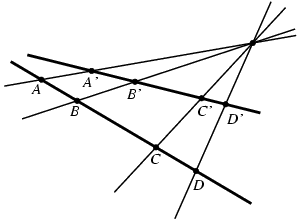The cross ratio

The cross ratio is of remarkable value in projective geometry, since it is invariant under perspective transformations. So, if you have four points A, B, C, and D on a line and centrally project them to four points A′, B′, C′, and D′ on another line, then the cross ratios of the two quadruples of points are identical.

Similarly, the cross ratio of four lines through a point P can be defined to be the cross ratio of four points that are the intersections of each line with another distinct line not going through the point P.

Now the definition of a Cayley-Klein geometry is easy. Choose a quadratic form

 x² + y² + cz² + exz + fyz = 0.

The zero set of this equation describes a (possibly complex) conic in the projective plane. This is the "fundamental object" of the geometry. Now measurements of angles and distances are defined as follows: For the distance between two points A and B take the line joining them. The intersection of this line with the fundamental object is two points X and Y. Calculate the cross ratio (A, B | X, Y). Take the logarithm of that number and call the result distance.

Angles are calculated in an analogous way. For the angle between two lines L and M first take their meet, that is, their point of intersection. The tangents through the meet that touch the fundamental object are two lines P and Q. Calculate the cross ratio (L, M | P, Q). Take the logarithm of that number and call the result angle. Usually, these two functions are multiplied by some cosmetic constants r and s in order to match the traditional definitions of measurements.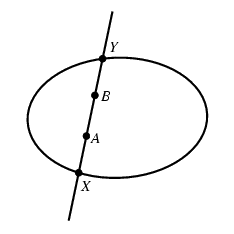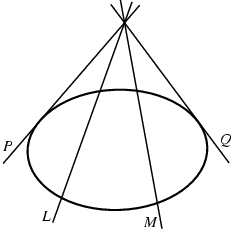dist(AB) = r·ln(A, B | X, Y) angle(LM) = s·ln(L, M | P, Q)

It may sound like magic, but this is all you have to know. Depending on the type of fundamental object that you have chosen, you get different kinds of geometry. Up to isomorphism there are exactly seven different types of geometries you obtain that way. The three most important choices for the fundamental conic are the following:

• The circle given by x² + y² - z²= 0: The resulting measurement corresponds to hyperbolic geometry.
• The degenerate conic described by x² + y² = 0: The resulting measurement corresponds to usual Euclidean geometry.
• The equation x² + y² + z² = 0 with no real solutions: The resulting measurement corresponds to elliptic geometry.

Two things are worth mentioning:

• Distances in Euclidean geometry need a little twist. The formulas of Cayley-Klein geometry will always produce a zero distance. This is due to the fact that in Euclidean geometry there is no "absolute" notion of distance. Each length has to be compared to a unit length. The right formulas are obtained immediately in the limit situation.

• The intersections and tangents in the above constructions do not necessarily have real coordinates. For instance, in the case of elliptic geometry the fundamental object has no real points at all. In this case the intersections of the fundamental object with a line are always complex.

The metric part of Cinderella is based on Cayley-Klein geometries. All calculations of lengths, angles, orthogonality, circles, etc. refer to a fundamental object.

We finally want show at least one effect that is caused by this general theory. We do this in order to give you a feeling for what complex numbers, cross ratios, and projective geometry have to do with measuring.

We consider the case of Euclidean geometry. There the fundamental object has the equation x² + y² = 0. Using complex numbers, this quadratic form can be factored into two linear forms: x² + y² = (1·x + i·y + 0·z) and x² + y² = (1·xi·y + 0·z). The points I := (1, i, 0) and J := (1, –i, 0) that occur in this formula play a special role in Euclidean geometry. They are not affected by any Euclidean transformation. In a very well defined way we can say that "Euclidean geometry is projective geometry together with I and J."

The points I and J are sometimes called the imaginary circle points, since they have a very special relation to circles: each Euclidean circle passes through I and J. To see this consider a general circle equation in homogeneous coordinates:

 x² + y² + cz² + exz + fyz = 0.

Now plug in the coordinates of I and J. Using the rules for calculating with complex numbers we observe that the circle equation is obviously satisfied. Thus we can say that a circle is a special conic that passes through I and J. With the notion of a circle it is easy to define what it means to have equal distances or equal angles. The remaining concepts of Euclidean geometry can be derived in a straightforward manner. A very good classical source on Cayley-Klein geometries can be found in [Kle28b]. A Modern treatment of these issues that also includes a broad introduction into projective Geometry may be found in [RGO09] and [RG11].

## The Principle of Continuity

It was mentioned in the preface of this manual that Cinderella uses some basic new methods to avoid inconsistent behavior. The geometric system we presented in the previous sections is a closed framework for doing geometry, including measurements. However, so far there is an element missing that is crucial for Cinderella: dynamics. Most other systems for interactive geometry, or parametric CAD, suffer from inconsistencies that come from an unsatisfactory treatment of the special effects of dynamics. For instance, consider the "theorem" that the angular bisectors of the sides of a triangle meet in a point. Every pair of lines has two angular bisectors that are perpendicular to each other. Depending on the choice of the angular bisectors, the above statement can be true or false. Now imagine you have constructed an instance of this "theorem" (i.e., you have chosen the correct bisectors). You start to drag the vertices, around and suddenly, without reason, one angular bisector flips to the other position and the "theorem" becomes false. Such a scenario can happen in any system that does not take extra measures to resolve the special problems from the dynamic aspects of geometry.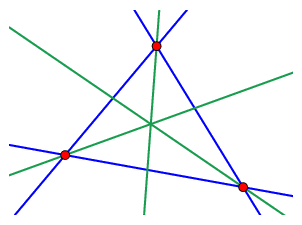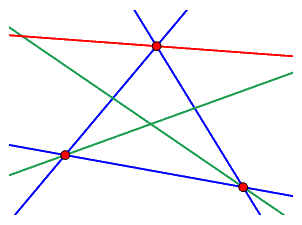The angular bisectors of a triangle can intersect… …or not.

Let us consider another small construction. Take two circles and one of their intersections. While you drag elements around, Cinderella has to decide for every mouse move which intersection you "mean."

It is a good first attempt to "trace" this point of intersection using the following rule: "Always take the intersection that is closest to the previous position," since this precisely reflects the definition of continuity. But how should we deal with vanishing intersections? Again it pays off having implemented everything in complex space. In Cinderella intersections never vanish; they can become complex, however. So we have to trace the intersections in complex space and use the above rule.

However, this is not enough. When you separate two circles that were previously intersecting, there is always a position in which the two points of intersection coincide. How can the points be distinguished in this situation? This time it is analytic function theory that rescues us. If "detours" through complex space are allowed, then there is always a path that avoids all degenerate situations. Again the whole approach is possible only because everything is embedded in a complex space.

Here is an approximate description of what happens while you drag the mouse from one position to another in the "move" mode of Cinderella. While you move a point from position A to position B:

• Cinderella generates a path from A to B through complex space that avoids all degeneracies.
• The dependent elements are traced through complex space.
• The number of intermediate steps on the path is adjusted according to the required accuracy.

For the tracing, Cinderella uses an adaptive step-width algorithm. You can imagine that while you drag the construction elements the mouse pointer leaves the "real screen" and walks through complex space.

Why all this effort? With these methods we can be sure that elements do not "jump around" for no reason. So when you start with a correct drawing of the angular bisectors theorem you will never be able to move it to a false position. The theory also forms the basis for reliable randomized theorem checking and for the loci and animation functions of Cinderella.

Contributors to this page: Richter and Kortenkamp .
Page last modified on Sunday 04 of September, 2011 [18:14:53 UTC] by Richter.